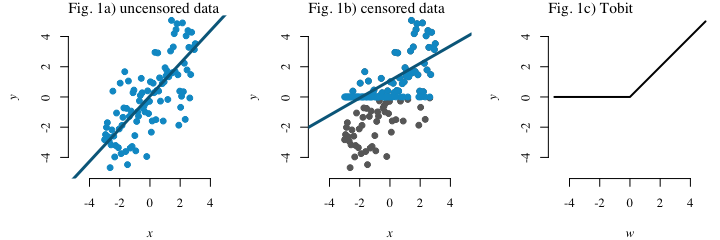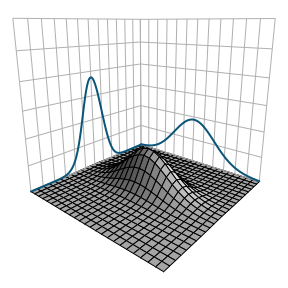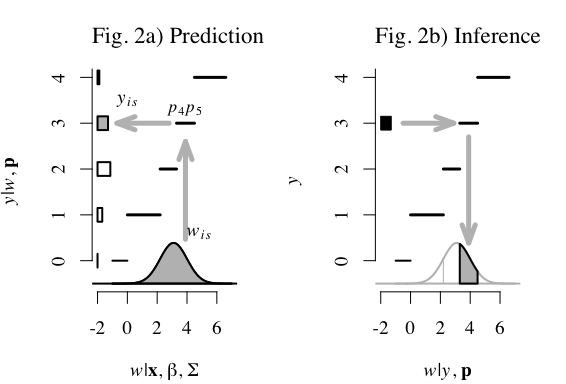# GJAM Explainer

Using a Bayesian hierarchical framework, the Generalized Joint Attribute Model (GJAM) fits individual species at the community scale, i.e., all species jointly, and admits biodiversity data that are…

1. multivariate
2. multifarious (measured in different
ways and on different scales)
3. mostly zeros
4. high-dimensional (thousands of species)
5. multi-trophic levels

In order to highlight key aspects, we show the logical progression from a simple linear model to a GJAM.

### Linear regression

$$y_{i} \sim N(\beta 'x_{i},\sigma^{2})$$

• We first simulate some uncensored data and show that a simple linear model fits the data well (Fig. 1a).
• However in ecology most response variables are censored (e.g., basal area, species counts).
• When we censor the data, the linear model has a poor fit (Fig. 1b).

### Censored regression

$$\begin{matrix} y_{i} = \left\{\begin{matrix}w_{i} \quad & if \enspace w_{i} > 0\\ 0 \quad & if \enspace w_{i}\leq 0\end{matrix}\right.\\ \ \\w_{i} \sim N(\beta 'x_{i},\sigma^{2})\end{matrix}$$

• Instead of relying on a linear model, we can use a tobit model, which works well for censored data (Fig. 1c).
• Here there is a latent (unobserved variable) $$w_{i}$$, which is linearly dependent on $$x_{i}$$.
• The observed response variable, $$y_{i}$$ is equal to $$w_{i}$$ when $$w_{i}$$ is greater than zero, and is otherwise zero.
• However, this model is univariate, and in ecology we are interested in modeling an entire community, with multiple response variables.### Multivariate tobit

$$\begin{matrix} y_{is} = \left\{\begin{matrix}w_{is} \quad & if \enspace w_{is} > 0\\ 0 \quad & if \enspace w_{is}\leq 0\end{matrix}\right. \\ \ \\w_{i} \sim MVN(\beta 'x_{i},\Sigma) \end{matrix}$$

• Therefore, we can extend the model to a multivariate tobit, where $$y_{i}$$ is a vector of responses: $$y_{i} = (y_{i1}, y_{i2},...,y_{iS})$$
• and $$\Sigma$$ is an $$S \times S$$ covariance matrix.
• However, this model only applies to continuous abundance data (e.g., basal area).### Generalized Joint Attribte Model (GJAM)

$$\begin{matrix}y_{is} = \left\{\begin{matrix}w_{is} \quad & if \enspace continuous \\ z_{is},w_{is} \in (p_{z_{is}},w_{z_{is} +1}] \quad & if \enspace discrete \end{matrix}\right. \\ \ \\w_{i} \sim MVN({\color{Green} \beta'} x_{i},{\color{Blue} \Sigma}) \times \prod_{s=1}^{S} I_{is} \end{matrix}$$

• there is a $$\beta$$ coeficient for each species and environmental covariate.

• the covariance $$\Sigma$$ represents the covariance between species beyond what has already been explained by the environmental covariates. It can include interactions between species, unacounted for environmental gradients, and other unexplained sources of error.

• The multivariate tobit framework can then be extended to accomodate multiple types of data.
• GJAM itegrates discrete and continuous data using censoring, where continuos observations are uncensored, and discrete observations are censored.
• Therefore, $$y_{i}$$ can include both continuous and discrete response variables. A partition, $$p_{is}$$ segments the real line into intervals than can be censored or uncensored. $$I_{is}$$ is an indicator function specifying whether $$w_{is}$$ is in the correct interval or not.
• As an example, Fig. 2 shows how GJAM works for discrete data.#### citation:

Clark, J.S., D. Nemergut, B. Seyednasrollah, P. Turner, and S. Zhang. 2017. Generalized joint attribute modeling for biodiversity analysis: Median-zero, multivariate, multifarious data, Ecological Monographs, 87, 34-56.

website